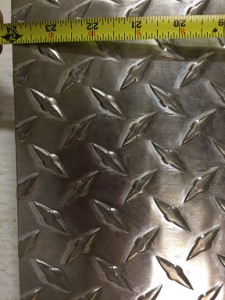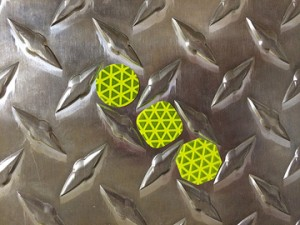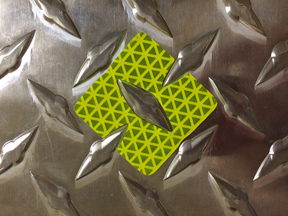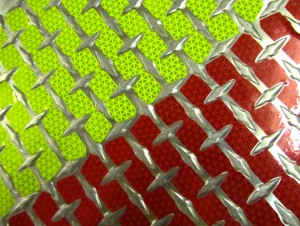# Estimating the Number of Reflective Dots or Shapes for Diamond Plate

How to Calculate the Number of Diamonds, Dots, Octagons, Overlays or Chevron Strips for Diamond Plate – www.reflectiveshapes.com

CLICK HERE To go to our online “Reflective Shape and Chevron Striping Calculator”.

The process of estimating how many reflective shapes to purchase to cover a given amount of diamond plate is a fairly simple process.  It involves measuring the size of the diamond plate area and then dividing the coverage per shape into the size.  The details for each of our reflective shapes is as follows –Measuring your diamond plate is the first step you will take regardless of which shape you choose.  You are going to want to calculate the area of diamond plate in both square inches and square feet.  To calculate square inches of area just measure the width and height of your diamond plate in inches and then multiply those two numbers together.  For example, a 24 inch wide x 36 inch high area of diamond plate contains 864 square inches.  Now to convert the 864 inches into square feet you simply divide by 144.  864 divided by 144 equals 6 square feet.

To summarize, inches of height times inches of width gives you the total square inches of diamond plate that you have. Dividing by 144 changes this number to square feet.

3/4″ DOTS, ROUNDED SQUARES AND OCTAGONSEach 3/4″ shape fits into a 1 square inch area of diamond plate.  It takes 144 – 3/4″ shapes to cover one square foot of diamond plate.  (12″ x 12″ = 144″)  A sheet of 100 – 3/4″ shapes will treat 100 square inches of diamond plate.  To calculate how many 100 shape sheets (dots, rounded squares or octagons) you would need you simply divide 100 into the square inches of diamond plate that you have to cover.

For example, say you have a 36″ x 48″ area of diamond plate to cover.  That is 1728 square inches of diamond plate.  If you divide 100 into 1728 you get 17.28 so you would need to purchase 18 sheets of dots.  9 would be red and 9 would be fluorescent lime since you are doing two colors to make your chevron pattern.  The 3/4″ shapes currently run \$10 per sheet so your cost would be \$180 plus shipping.

DIAMOND PLATE OVERLAY APPLIQUESOur diamond plate overlays cover a 4 square inch section of diamond plate each.  They come 1 square foot or 36 shapes to a sheet for \$18.  Therefore each sheet covers 1 square foot.  To calculate how many sheets you need, simply measure the square footage of the area you want to cover.  That will be how many square feet of material you need.  To do two colors simply divide by 2.

For example, say you have a 36″ x 48″ area of diamond plate to cover.  That is 1728 square inches and if you divide by 144 you get 12 square feet.  Or just multiply 3 x 4 sf to get the 12.   12 sf x \$18 = \$216. This is a little more that you would spend on dots but you are going to get more coverage and your application time will be less.

CHEVRON STRIPS FOR DIAMOND PLATEOur chevron strips cover 6 square inches of diamond plate per strip.  The strips are sold by the square foot (24 strips).  To get the number of square feet you need you would simply measure your diamond plate in square feet and order that amount.

For example, a 36″ x 48″ area of diamond plate is 3 feet x 4 feet and when you multiply 3 x 4 you get 12 square feet.  You would need 12 square feet of strips to cover that amount of diamond plate.  Each square foot of strips is \$18 so 12 x \$18 = \$216 plus shipping.  You would order 6 square feet of red and 6 square feet of fluorescent lime.

Our chevron strips come in 4 styles, A, B, C and D. This allows for the various spacings for different diamond plates.  Our sample pack contains all 4 types and you can test for a perfect fit.

DIAMOND TABS FOR DIAMOND PLATEOur newest shape is our “Diamond Tabs” for making diamond plate reflective. Measuring for diamond tabs is the same as for our overlay or strips. Simple measure length times width of your area or areas and get a total square feet for your job. You need a sheet for every square foot you want to cover. Half red, and half lime. Diamond Tabs cover more of the flat area and are there for brighter and have a better overall appearance. Each square foot coverage sheet runs \$20.